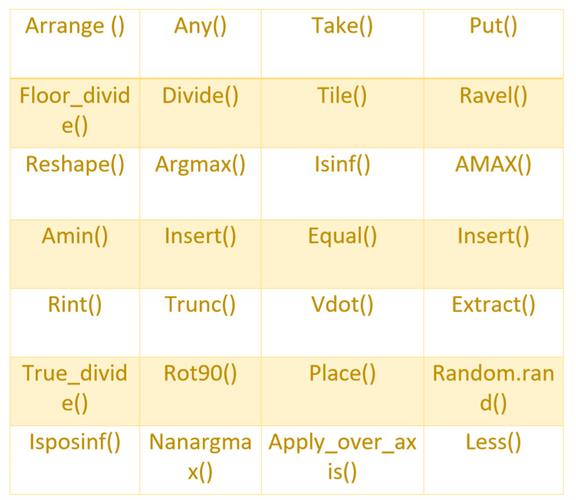Loading, please wait...# NUMPY - DATA SCIENCE ( PART 2 )

Sep 23, 2019 Data Science, September Series, Python, 3134 Views

Numpy provides a quality performance array processing package with a multidimensional array object and tools for working with these arrays. It also contributes to scientific uses, by enabling efficient multi-dimensional container for generic data and scientific computing with python.

## GENERAL - METHODS IN NUMPY## ARRAY ( NUMPY WAY )

In Numpy the arrays are of all same type usually indexed by a tuple of positive integers. The dimension of the array are called the rank of the array. An array in Numpy class is usually called as ndarray.

### Creation of array in Numpy ( Python )

``````import numpy as np
arr = np.array([10, 20, 30])

# Creation of array with rank 1 Array
print("Array with Rank 1 = \n",arr)

# Creation of array with rank 2 Array
arr = np.array([[10, 20, 30],[40, 50, 60]])
print("Array with Rank 2 = \n", arr)

# Creation an array from tuple
arr = np.array((10, 30, 20))
print("\nArray created using passed tuple = \n", arr)``````

### Operations - Basic Operation is Array using Numpy

``````import numpy as np

# Defining Array 1
a = np.array([[10, 20],[30, 40]])

# Defining Array 2
b = np.array([[40, 30],[20, 10]])

# Adding 1 to every element
print ("Adding 1 to every element:", a + 1)

# Subtracting 2 from each element
print ("\nSubtracting 2 from each element:", b - 2)

# sum of array elements
# Performing Unary operations
print ("\nSum of all array elements: ", a.sum())

# Performing Binary operations
print ("\nArray sum:\n", a + b)``````

### Conclusion :

The above was an abstraction to shoe the use of Numpy in Data Science, but it needs to be emphasized more understand it in a more clear way, to know more the below-attached section is advised to refer.

### References:

https://numpy.org/

https://www.python-course.eu/numpy.php

https://docs.scipy.org/doc/numpy/reference/